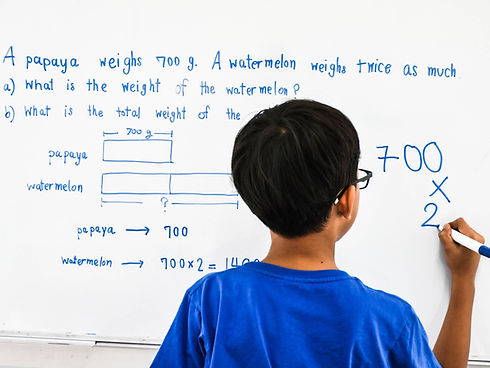top of page# ​

Math Word Problem Scenarios

Our Math Scenarios class aims to teach children how to successfully navigate mathematical word problems from 4th to 9th Grade.

​It is common for children to recoil when confronted with word problems.  This is because, in addition to testing a child’s computational skills, word problems test their reading comprehension.  If a child cannot understand the problem that is being posed, they will be unable to represent these word problems as equations and diagrams., Our teachers understand this fact and aspire to support children in the synthesis of the reading and math skills necessary to successfully solve word problems.  Your child will learn to translate word problems into actionable math equations.

This 10-week course will strengthen students’ ability to tackle word problems by…

• Enhancing their  knowledge of key mathematical terms/concepts

• Learning key terms to translate them into the proper mathematical action.

• Review math concepts that are connected and build on each other

• Annotate, identify key values, and recognize vocabulary and phrases in word problems.

• Connecting mathematical concepts to the real world

• Honing on logic and critical thinking as they work through single and multi-step word problems using addition, subtractions, multiplication, division of whole numbers, decimals, fractions and Geometry.

bottom of page